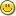### Euphoria Ticket #480: Sequence routines foldl and foldr

These methods combined a sequence into 1 value based on the output of a user supplied routine. For example:

```function subt(integer a, integer b)
return a-b
end function

? foldl({1,2,3}, routine_id("subt")) --> -4
? foldr({1,2,3}, routine_id("subt")) -->  0
```

This is an ultra simple example. You can imagine more complex ones. foldl took 1 and 2, giving it to subt(), the result of that and 3. foldr would start at the right side, thus 3 and 2 goes to subt(), then the result of it and 1.

For more details: wikipedia:Fold_(higher-order_function)

#### Details

Type: Severity: Category: Feature Request Normal Library Routine unknown New 4450 none 4.0.2

#### 1. Comment bymattlewis Dec 02, 2010

That's very terse. What about fold_left and fold_right?

#### 2. Comment byjeremy Dec 02, 2010

Doesn't matter to me, I was just taking the names from other languages I've used. Another really common name is reduce, but it doesn't lend itself to left/right very easily.

#### 3. Comment byjimcbrown Dec 02, 2010

Isn't stdseq:apply() the same as fold_left() / foldl() ?

#### 4. Comment byjimcbrown Dec 02, 2010

Uh, nvm, it's not.

#### 5. Comment byDerekParnell Dec 02, 2010

Don't we already have something like this?

And what is the bug you are fixing by adding this functionality to the library?

#### 6. Comment byjeremy Dec 02, 2010

No. Apply does this:

```function add_10(integer a)
return a + 10
end function

-- 11, 12, 13
```

i.e. it returns a sequence the same size as the source. It does not combined the results of the user routine.

#### 7. Comment byjeremy Dec 02, 2010

Should have been a feature request, and it's slated for 4.1.

If we have it, please tell me where it is. I think we missed it.

#### 8. Comment byDerekParnell Dec 02, 2010

Are there any obvious examples of why someone would need this function?

#### 9. Comment byjimcbrown Dec 02, 2010

```function wrapped_or_bits(object x, object y)
return eu:or_bits(x, y)
end function
function or_all(sequence flags)
return fold_left(flags, routine_id("wrapped_or_bits"))
end function
```

#### 10. Comment bymattlewis Dec 02, 2010

It's useful as an iterative application of something like apply(). The left vs right could be useful for non-commutative operations, though identical results could be achieved, I suppose by using reverse() on the sequence first.

#### 11. Comment byjeremy Dec 02, 2010

Some languages do not have foldl/r, they just have fold. In those languages you do just that, use fold(reverse(...), ...)

#### 12. Comment byDerekParnell Dec 02, 2010

Hmmm ... I see your point but I think most people would prefer a bit more speed with such simple tasks. The 'or_all' example above is almost the slowest way of achieving that result.

#### 13. Comment byjeremy Dec 02, 2010

Many times convenience is better than speed. Optimizations later on, esp with things such as seeing a routine_id() is constant, and call_proc/func should just call the func directly would make operations like this just as fast and convienient.

Further, we have map (mapping) and filter. The mainstays of a functional language are map, filter and fold. The other two feel lonely#### 14. Comment byjimcbrown Dec 02, 2010

I guess you could use transform() to do this, sort of, but it's not really ideal:

```function or_all(sequence source_data)
integer rid = routine_id("do_or_bits")
sequence s = transform(source_data, repeat(rid, length(source_data)))
return s
end function
function do_or_bits(sequence s)
s = or_bits(s, s)
return s[2..\$]
end function
```

or more generically

```integer call_rid = NO_ROUTINE_ID
function fold_left(sequence source_data, integer rid)
call_rid = rid
sequence s = transform(source_data, repeat(helpfold_rid, length(source_data)))
return s
end function
function helpfold(sequence s)
if length(s) < 2 then
return s
end if
s = call_func(call_rid, {s, s})
return s[2..\$]
end function
constant helpfold_rid = routine_id("helpfold")
```

Of course, this implementation is much easier

```function fold_left(sequence source_data, integer rid)
object x
if not length(source_data) then
return ""
end if
x = source_data
for i = 2 to length(source_data) do
x = call_func(rid, {x, source_data[i]})
end for
return x
end function
```

#### 15. Comment byjeremy Jan 03, 2011

Assigned to 4.0.1 milestone